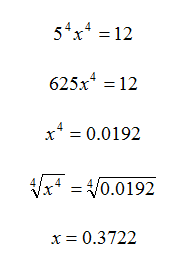Method 2
Example 2

Solve the equation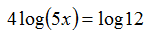Step 1. Use Power of a Power Property to convert to a single log.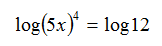Step 2. Use the inverse operation of exponents on both sides of the equals sign. Since the common log has a base of 10, use an exponential base of 10.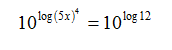Step 3. Since logs and exponents are inverses, the exponent base and the log base cancel.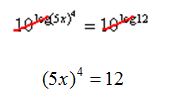Step 4 . Solve for x.